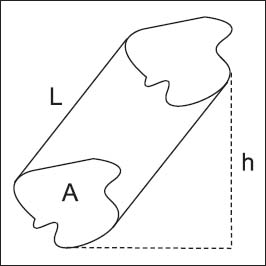NextPrevious

# What are geometric transformations?

Geometric transformations are rules that mean there is a one-to-one correspondence between two sets of points in two figures. A geometric transformation changes the plane or three-dimensional space. Each transformation can be defined by thinking about where each point is “taken,” with a point said to be “taken to its image.”in this irregular prism shape, the lateral area equals the perimeter of shape A multiplied by L, and the surface area equals the lateral area plus two times the area of shape A.

One way to visualize a transformation is to think of expanding, shrinking, or moving one figure to get a second figure. Depending on the transformation, the image will either be congruent (equal) to the original image (called the identity transformation), similar but not necessarily equal, or will not resemble the original at all.

Close

This is a web preview of the "The Handy Math Answer Book" app. Many features only work on your mobile device. If you like what you see, we hope you will consider buying. Get the App